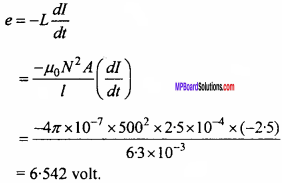## MP Board Class 12th Physics Important Questions Chapter 6 Electromagnetic Induction

### Electromagnetic Induction Important Questions

Electromagnetic Induction Objective Type Questions

Question 1.
Choose the correct answer of the following:

Question 1.
The SI unit of magnetic flux is :
(a) Weber
(b) Gauss
(c) Oersted
(d) Tesla
(a) Weber

Question 2.
The flux linked with a coil can be charged by :
(a) Keeping the coil in a time varying magnetic field
(b) Rotating the coil in a magnetic field
(c) By charging area of coil in the uniform magnetic field
(d) All the above.
(d) All the above.

Question 3.
The direction of induced current can be determined by :
(a) Lenz’s law
(b) Flemming’s left hand rule
(c) Right hand palm rule
(d) Ampere’s swimming rule.
(a) Lenz’s law

Question 4.
In the phenomenon of elctro magnetic induction :
(a) Electrical energy is transformed into mechanical energy
(b) Mechanical energy is transformed into electrical energy
(c) Electrical energy is transformed into thermal energy
(d) Mechanical energy is transformed into thermal energy.
(b) Mechanical energy is transformed into electrical energy

Question 5.
The self inductance of a coil does not depend upon :
(b) Number of turns in the coil
(c) Lenght of coil
(d) Current through the coil.
(d) Current through the coil.Question 6.
If a core of soft iron is placed between the coil then their mutual inductance :
(a) Decreases
(b) Increases
(c) Remain unchanged
(d) Nothing can be said.
(b) Increases

Question 7.
The eddy current are used in :
(a) Electrolysis
(b) Making a galvanometer dead beat
(c) Electroplating
(d) Increasing the sensitivity of galvanometer.
(b) Making a galvanometer dead beat

Question 2.
Fill in the blanks :

1. Lenz’s law is used to find out direction of ……………
2. The SI unit of magnetic flux is ……………
3. The core of transformer is made laminated to reduce the loss of energy due to …………… currents.
4. To make the moving coil galvanometer …………… its coil is wound on the aluminium frame.
5. Magnetic flux is a …………… quantity.

1. Induced current
2. Weber
3. Eddy
5. Scalar.

Question 3.
Match the Column :
I.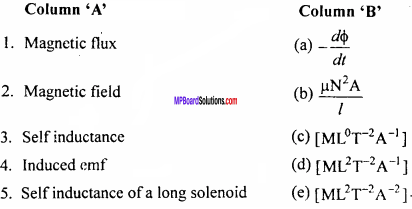1. (d)
2. (c)
3. (e)
4. (a)
5. (b)

II.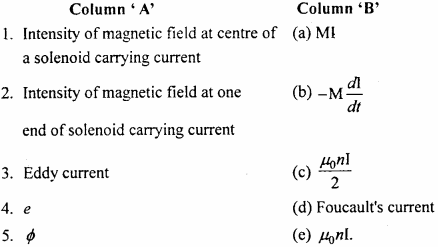1. (e)
2. (c)
3. (d)
4. (b)
5. (a)

Question 4.
Write the answer in one word/sentence :

1. What is the unit of magnetic flux?
2. What is unit of coefficient of self induction?
3. A straight conductor of length ‘l’ moving with a velocity of ‘V’ in a uniform magnetic field ‘B’ Perpendicular to it. Write the
4. formula for induced e.m.f. in it.
5. Write relation between Henry and Micro Henry.
6. Write the principle of alternating current generator.
7. Induced electric field is conservative field or non conservative field.
8. In which form the energy is stored in a coil?
9. By which law we determine the direction of induced e.m.f.?

1. Weber
2. Henry
3. e = Blv
4. 1H = 106pH
5. Electro magnetic induction
6. It is Non conservative field
7. Magnetic energy
8. Lenz’s law.

Electromagnetic Induction Very Short Answer Type Questions

Question 1.
When induced current flow in a closed circuit?
When there is change in magnetic flux in a closed circuit, then induced current flow over it.

Question 2.
If a iron core is kept at the centre of a coil, then what will be the effect on coefficient of self induction?
Coefficient of self induction will increase.

Question 3.
Lenz’s law is accordance to which law?
Law of conservation of energy.

Question 4.
If any metal piece is kept in varying magnetic field, will eddy current generate in it?
Yes, eddy current generate in the metallic piece.Question 5.
Why coil with in the resistance box is double coiled?
To avoid self – induction phenomenon.

Question 6.
When the magnitude of magnetic flux become maximum and minimum?
When magnetic field is perpendicular to the plane, the magnetic flux is maximum and when it is parallel, its value is minimum.

Electromagnetic Induction Short Answer Type Questions

Question 1.
What is magnetic flux? Write its SI units.
The number of magnetic lines of force passing normally through any area in a magnetic field, is called magnetic flux linked with that area. It is denoted by ϕ(phi).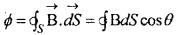Where, $$\vec { B }$$ is the magnetic field, $$\vec { dS }$$ is the normal to the surface and θ is the angle between $$\vec { B }$$ and normal to surface. SI unit of fluxs weber.

Question 2.
Write Lenz’s law of electromagnetic induction.
According to this law, the nature of induced e.m.f. is such that it opposes the cause which produces it. With the help of this law, the direction of induced e.m.f. can be determined.
∴ e = $$\frac {dϕ}{dt}$$
– ve sign is according to Lenz’s law.

Question 3.
A bird sitting on a wire carrying current at high voltage, flies away. Why?
As soon as the current flows through the wire, an induced current is produced in the body of the bird, the direction of the current in the wings are opposite. Hence, they are repelled and the bird flies away.

Question 4.
In the given figure, which plate of the capacitor AB is positive and which plate is negative?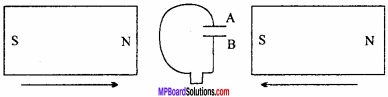According to Lenz’s law induced current flows from B to A, therefore plate A is positive and plate B is negative.

Question 5.
A metallic chain touching the ground is attached with the truck carrying explosive matter. Why?
During the motion of the truck, the axle cut the magnetic flux of the earth, which causes an induced e.m.f. set up across its axle. This induced charges are earthed by the chain. Which prevent the explosive from the fire.

Question 6.
In metre bridge experiment, the battery’ key is pressed first then galvanometer. Why?
If the galvanometer key is pressed first and then battery key, an induced current will be produced in the galvanometer circuit and galvanometer will give deflection even at the position of null point. Thus, the battery key is pressed first.

Question 7.
The resistances in the resistance box are double coiled. Why?
In the double coil, the current flows in opposite directions, thus the magnetic fields produced cancel each other and hence induced current is not produced.

Question 8.
When any electric circuit is suddenly cut off, then sparks take place. Why?
When the switch is off, the magnetic flux linked with the circuit becomes zero suddenly. Therefore, a strong induced current flows through the circuit in the direction of main current, which causes a high potential difference across the terminal and due to electric discharge, sparks are seen.Question 9.
What is mutual induction?
When the current changes in a coil, then the flux linked with another coil placed near the first changes, hence an induced current is produced in another coil. This phenomenon is known as mutual induction.

Question 10.
A coil in magnetic field is moved

1. Rapidly and
2. Slowly.

Are the e.m.f. and work done in the two cases equal or not?
No, the e.m.f. induced in the first case will be larger due to larger rate of change of flux. Obviously, in the second case, the rate of change of flux is slow and hence induced e.m.f will be smaller.

Question 11.
Two coils A and B are kept perpendicular to each other as shown in figure. If flow of current in any one coil is change will induced current will generate in the second.
When current is flows in use one coil, the induced flux produce will be parallel to second coil and there will be no change in magnetic flux. Hence no induced current will be produced in second coil.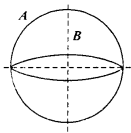Question 12.
A ring is hanging in a wall of room. When the north pole of the magnet is brought closer to the ring. Then what will be the direction of induced current if seen from the direction of magnet?
The face of the ring which is toward the north pole of the magnet, in.it the direction of induced current will be anti clockwise. Hence this face become magnetic north pole and resist the magnetic pole to come near it.

Question 13.
Three coils are arranged as shown in the figure :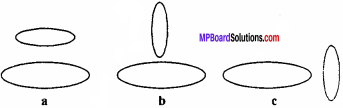In which coil mutual induction will be maximum?
In figure (a), the mutual induction will be maximum before the magnetic lines of force passing in figure (a) is more in comparison to other figure.

Question 14.
What is electromagnetic induction? State Faraday’s laws of electromag neticinduction.
Or
Write down the laws of electromagnetic induction. Find out the expression for the induced electromotive force.
When a magnet and a conducting coil are in relative motion, an e.m.f. is induced in the coil. This e.m.f. is called induced e.m.f. If the coil is closed, a current flows in it, then this current is called induced current and the phenomenon is called electromagnetic induction.

1. Whenever there is a change in magnetic flux linked with an electrical circuit, an e.m.f. is induced in the circuit, therefore an induced current flows in it. The induced current losts so long as there is change in flux.

2. The induced e.m.f. is directly proportional to the rate of change in magnetic flux.

Formula for induced e.m.f.:
Let Φ1 weber be the flux linked with a closed electrical circuit at any instant of time t1. After time ∆t i.e., at time t2 seconds, let the flux be Φ2 weber.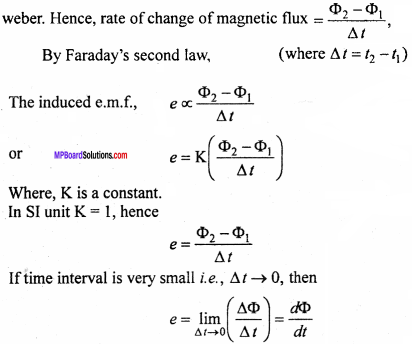This is the required formula.
Hence, the induced e.m.f. is directly proportional to the rate of change of flux.

Question 15.
Write Lenz’s law and explain how it can be used to determine the direction of induced current?
Lenz’s law:
According to this law, the direc¬tion of induced current is such that it opposes the very cause producing it. With the help of this law, the direction of induced current can be determined as follows :

1. When N – pole of a bar magnet is brought closer to a coil, a current is induced to develop N – pole on the adjacent face of the coil to oppose the motion of N – pole towards the coil. This means the induced current must be anticlockwise as seen from the magnet, [see Fig.(a)].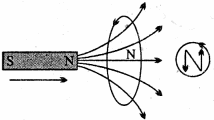2. When N – pole of the bar magnet is taken away from the coil, an induced current flows in the coil such that the face of the coil near the magnet becomes south pole which attracts the north pole and thus opposes the cause producing it (i.e., opposes the motion of the magnet away from the coil). So, the direction of the induced current in the coil must be clockwise as seen from the magnet, [see Fig. (b)].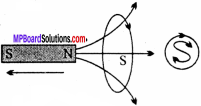Question 16.
Explain that Lenz’s law is in accordance with the law of conservation of eaefgy.
When a magnetic north pole is brought near a plane of a coil, the magnetic pole induced in that plane is also north pole causing a repulsive force on the north pole of the magnet. Therefore, work will have to be done against this repulsive force to bring the magnet’s north pole closer to the coil. The work done (mechanical energy) is transformed into electri¬cal energy causing a current induced in the coil.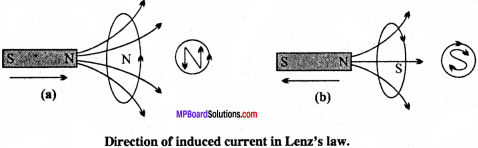But, on moving the north pole of the magnet away from the coil, a south pole is developed on the face of the coil and an attractive force is developed on the face of the magnet. So, work has to be done in moving the magnet away from the coil. This results in flow of induced current in reverse direction. Thus, Lenz’s law is in accordance with the law of conservation of energy.

Question 17.
Explain self – induction and demonstrate the phenomenon by an experiment.
Self – induction:
During the motion of the truck, the axle cut the magnetic flux of the earth, which causes an induced e.m.f. set up across its axle. This induced charges are earthed by the chain. Which prevent the explosive from the fire.

Experiment:
The circuit of the experiment is shown in the figure. It consists of an insulated copper coil L, wound on soft iron core. Cell E, rheostat Rh and a tapping key K are connected in series. A bulb B is connected in parallel with the coil. Now, the key is closed, the bulb glows slowly, then becomes bright and when it is opened, the bulb flashes bright and then goes off.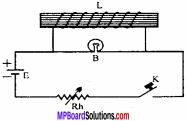As the key is pressed, the flux linked with coil changes and an induced current flows in the opposite direction of main current, because of this the current grows slowly in the circuit.But when the key is opened, the flux suddenly decreases to zero, therefore a strong induced current flows in the same direction of circuit current makes the bulb very bright for a moment.

Question 18.
What is self – induction? What is meant by self-induction of coil? Explain. WritgTts unit.
Self – inductance:
When the current flowing through a circuit, an induced e.m.f. is set up in the same circuit, which opposes the main current. This phenomenon is called self – induction.
When the current increases in the circuit, induced current flows in the opposite direc¬tion and when the current decreases in the circuit, the induced current flows in the same direction of circuit current.
Let ϕ be the flux linked with a circuit when a current (l) flows through it. Then,
ϕ ∝I
or ϕ = LI …(1)
Where, L is a constant, called self – inductance of the coil.
Now, if I = 1, then by eqn. (i), we get
ϕ = L
Thus, the self-inductance of a coil is numerically equal to the magnetic flux linked with the coil, produced by unit current.
By Faraday’s law, e = –$$\frac {dϕ}{dt}$$
Putting the value of ϕ from eqn. (i), e = –$$\frac {d(LI)}{dt}$$
or e = -L$$\frac {dI}{dt}$$
If $$\frac {dI}{dt}$$ = 1
Then, e = – L
Therefore, the self – inductance of a coil is numerically equal to the opposing e.m.f. produced in the coil due to a unit rate of change of current in the circuit.
Unit : Unit of self-inductance is henry (H).Question 19.
Explain mutual inductance. Give its unit. On what factors does it depend?
or
Write down the definition, unit and dimensional formula of mutual inductance.
Mutual inductance is defined in two ways :
1. Let the flux linked with secondary coil be ϕ due to current l in primary coil.
∴ ϕ ∝ I
or ϕ = MI
Where, M is constant called mutual inductance.
If l = 1, then ϕ = M.
Hence, mutual inductance is defined by numerical value of flux linked with secondary coil, with unit current flows through primary coil.

2. When current changes in primary coil, then flux changes in secondary coil. Thus, by second law of Faraday
Induced e.m.fi, e = –$$\frac {dϕ}{dt}$$
or e = –$$\frac {d(MI)}{dt}$$
or e = ML$$\frac {dI}{dt}$$
If $$\frac {dI}{dt}$$ = 1
Then, e = – M
Thus, the mutual inductance of two coils is numerically equal to the induced e.m.f. set up in secondary coil at that moment, when the rate of change of current in primary coil is unity.

Unit : Its unit is Henry in SI system.
Dimensional formula :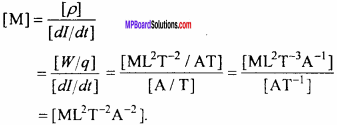It depends upon:

1. Number of turns of both the coils : As the number of turns increases, mutual inductance also increases.
2. Area of cross – section of both the coils : As the area increases, the mutual inductance increases.
3. Material of core : In presence of soft – iron core, the inductance is greater than that of air.

Question 20.
Give differences between self – induction and mutual induction.
Difference between self – induction and mutual induction :
Self – induction:

• This phenomenon happens in the same coil when the current flowing through the coil changes.
• One coil is used in this phenomenon.
• Induced current affects the main current.

Mutual induction:

• This phenomenon happens in the another coil placed nearby a coil in which the current is changing.
• Two coils are used in this phenomenon.
• The induced current produced in the second coil, therefore main current is not affected.

Question 21.
What are eddy currents? Show an experiment to demonstrate eddy currents.
Or
What are eddy currents? What are their disadvantages ? Write any two uses of eddy currents.
Eddy currents:
When a metallic plate is moved in a magnetic field or placed in a changing magnetic field, then flux linked with the conductor changes, hence an induced current is produced in the plate. This induced current is called eddy current.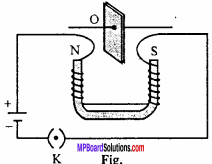Demonstration:
A rectangular copper plate is free to oscillate about a horizontal axis, passing through O. It is placed between the pole pieces of an electromagnet NS. Oscillate the plate, when no current is flowing in the circuit, the plate will oscillate for a long time and due to air resistance finally its amplitude of oscillation decreases.

Now, oscillate and pass the current. It is observed that the oscillations of the plate will be damped soon. Just as eddies are produced on water surface, the change of flux through the metal plate develops induced current known as eddy currents.

Uses : Eddy currents are used in :

1. Making a galvanometer dead beat.
2. Induction furnace.
3. Electric break.
4. Induction motor, etc.

Production of eddy currents causes loss of electrical energy in the form of heat. In order to prevent this, soft-iron core is laminated in a transformer. Lamination increases resistance and decreases eddy currents. So, there is very little dissipation of electrical energy in the form of heat.Question 22.
The self – inductance of two coils P and S are L1 and L2 respectively. If the coupling between them is ideal, then prove that the mutual inductance between these coils is M = $$\sqrt { { L }_{ 1 }{ L }_{ 2 } }$$
Let coil P is having N1 total number of turns, length of coil is l and I is the current flowing.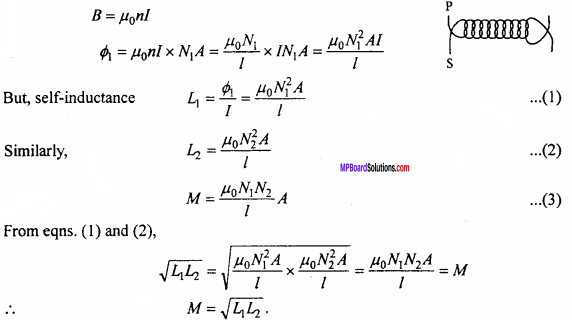Question 23.
Obtain an expression for the self – inductance of two coils when they are joined in parallel.
Consider two inductors of self – inductance L1 and L2and joined in parallel.
If I1 and L2, be the current flow through L1and L2 respectively, then
I = I1 + I2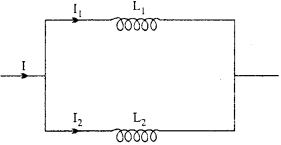Where 1 is the total current in the circuit differentiating both sides, we get
$$\frac {dI}{dt}$$ = $$\frac { { dI }_{ 1 } }{ dt }$$ + $$\frac { { dI }_{ 2 } }{ dt }$$ … (2)
In parallel combination, the induced e.m.f, across each coil remains the same.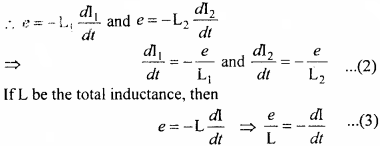Putting the value of $$\frac {dI}{dt}$$ From eqns. (1) and (3), we get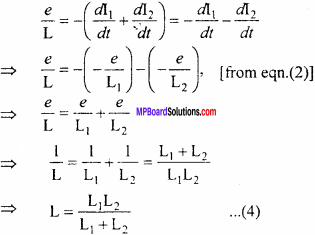Equation (4) is the required expression

Electromagnetic Induction Long Answer Type Questions

Question 1.
Derive an expression for mutual inductance ¡n between two circular plane coils. Write the factors affecting mutual inductance between circular plane colts.
Consider two circular plane coils P and S kept coaxially close to each other. Thenumber of turns in the primary coil P is n1 and in the secondary coil S is n2. The radius of primary coil P is r1 and that of the secondary coil is r2. Due to flow of current l in the primary coil, the magnetic field produced at its centre is
B = $$\frac { { \mu }_{ 0 } }{ 4\pi } \frac { 2\pi { n }_{ 1 }I }{ { r }_{ 1 } }$$ = $$\frac { { \mu }_{ 0 }{ n }_{ 1 }I }{ 2{ r }_{ 1 } }$$
The magnetic flux linked with secondary coil S due to this magnetic field.
ϕ = nBA
=n1 $$\frac { { \mu }_{ 0 }{ n }_{ 1 }I }{ 2{ r }_{ 1 } }$$ πr22 … (1)
But, ϕ = MI … (2)
From eqns. (1) and (2),
$$\frac { { \mu }_{ 0 }{ n }_{ 1 }{ n }_{ 2 } }{ 2{ r }_{ 1 } }$$.πr22
If some other medium is placed inside the coils in place of air or vacuum of perme – ability µ, then
M = µ$$\frac { { n }_{ 1 }{ n }_{ 2 } }{ 2{ r }_{ 1 } }$$.πr22 … (4)

The mutual inductance between circular plane coils depends on following factors :

1. The mutual inductance increases on increasing the number of trims in primary coil and secondary coil.
2. On increasing the radius of primary coil r1, the mutual inductance decreases.
3. On increasing the area of secondary coil, mutual inductance increases.
4. If a medium other than air is kept between the coil Mincreases.

Question 2.
Derive an expression for self – inductance of a long solenoid. On what factors does it depend?
Expression for self – inductance of a long solenoid:
Let the length and radius of a solenoid be l and r respectively. Also n be the number of turns per unit length.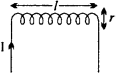∴ Area of cross – section, A = πr2
and total number of turns = nl = N.
If current l flows through the solenoid, then the intensity of magnetic field inside the solenoid will be given by
B = µ0nI, [∵B = $$\frac { { \mu }_{ 0 } }{ 4\pi }$$2πnl(cos00 – cos1800)]
(for unit length if l > >r)
Where, µ0 is the permeability of the medium.
∴ Magnetic flux linked with the total length of solenoid.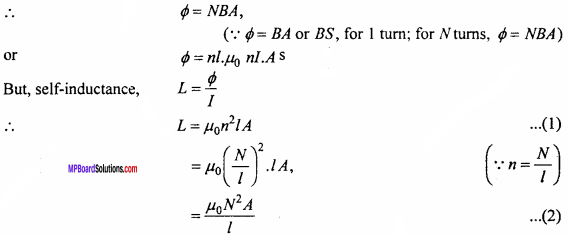If the permeability of soft – iron core inside the solenoid is µr, then
L = $$\frac { { \mu }_{ 0 }{ \mu }_{ 1 }{ N }^{ 2 }A }{ l }$$ … (3)
Equation (3) is the expression for self – inductance of a long solenoid. From this equation, it is clear that the self – inductance of a solenoid depends upon the following :

1. Area of cross – section of solenoid or radius of the solenoid : The self – inductance of a solenoid increases with radius and hence with the area of cross – section.

2. No. of turns : Self – inductance of a solenoid increases with number of turns.

3. Length of the solenoid : Self – inductance of a solenoid decreases on increasing the length of the solenoid.

4. Relative permeability of the core : The self – inductance of a solenoid increases on placing a core of higher permeability. This is the reason, that self-inductance of a solenoid with soft – iron core is greater than that of air core solenoid.

Equation (3) can be written in terms of radius as follows :
L = $$\frac { { \mu }{ N }^{ 2 }\pi { { r }^{ 2 } } }{ l }$$Question 3.
Obtain an expression for mutual inductance of two long solenoids. What are the factors on which mutual inductance depends?
Let S1, and S2 be the two long coaxial solenoids such that S1, is completely surrounded by S2. The lengths of both the solenoids are l. n1 and n2 are the number of turns per unit length respectively.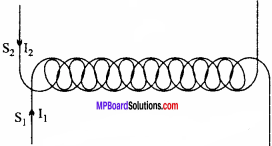Case :
1. Let S1 be taken as primary and S2 as secondary solenoid. If I1 is the current flowing through S1 then the intensity of field inside S1 will be
B1 = μ0n1I1
∴The magnetic field B1 will superpose on solenoid S2 and hence, magnetic flux linked with S2 per turn = B1A. Where, A is the area of cross – section of S1.
Hence, net flux associated with S2 will be
Φ21 = n2lB1A
Where, n2l is the total number of turns in solenoid S2 and Φ21 is magnetic flux on S2 due to S1
Substituting the value of B, from eqn. (1), we get
Φ21 = μ0n1I1n2A = μ0n1n2lAI1 … (2)
But, Φ21 = M21I1 … (3)
From eqns. (2) and (3),
M21 = μ0n1n2Al
This is the equation of mutual inductance of two solenoids. … (4)

2. Let S2 be taken as primary and S1 as secondary. If I2 is the current through S2, then the intensity of field inside S2 will be
B2 = μ0n2I2 … (5)
∴ The magnetic field of S2 i.e., B2 will superpose on solenoid S, and hence, magnetic flux linked with each turn of S1 = B2A.
Hence, the total flux associated with S1
Φ12 =n1lBn2A … (6)
Where, n1l is the total number of turns of solenoid S1, and Φ12 is magnetic flux on S1, due to S2.
Putting the value of B2 from eqn. (5), we get
Φ12 = μ0n2I2An1l = μ0n1n2lAI2 … (7)
But, Φ12 = μ12I2 … (8)
From eqns. (7) and (8), we have
M12 = μ0n1n2Al … (9)
From eqns. (4) and (9), we have
M21 = M12
This is called reciprocity theorem.
It is clear that the mutual inductance of two solenoids remains the same whether cur¬rent flows in one or the other.
Thus, mutual inductance of two long solenoids is given by.
M = μ0n1n2Al .
M = μ0μr$$\frac { { N }_{ 1 } }{ l } \frac { { N }_{ 2 } }{ l }$$Al
μ0μr$$\frac { { N }_{ 1 } }{ l } \frac { { N }_{ 2 } }{ l }$$Al

Mutual inductance depends upon :
1. Number of turns of both the coils : As the number of turns increases, the mutual inductance also increase.

2. Area of cross – section of both the coils : As the area increases, the mutual inductance increases.

3. Material of core : If the permeabilities of the cores in the solenoid are large, then mutual inductance increases. In presence of soft – iron core, the inductance is greater than that of air.

4. Length of the solenoid : For larger solenoids, mutual inductance is less.

Electromagnetic Induction Numerical Questions

Question 1.
The magnetic flux linked with a closed loop is given by the equation ϕB = 6t2 + 7t +1, where ϕB is in milliweber and t is in second. Find the electromotive force induced at time t = 2 second.
Solution:
Formula: e = – $$\frac {dϕ}{dt}$$
Given : ϕ = 6t2 + 7t +1
∴ = – latex]\frac {d}{dt}[/latex](6t2 + 7t +1) = – 12t – 7
At t = 2 sec,
e = – 12 x 2 – 7
= – 31 milli volt.

Question 2.
An aircraft with a wing span of 40 m flies with a speed of 1080 km hr-1 towards East where vertical component of Earth’s magnetic field is 1.75 x 10-5 tesla. Find the e.m.f. developed between the tip of the wings. (NCERT)
Solution:
l = 40m, Bv, Bv = 1.75 x 10-5T V = 1080$$\frac {Km}{hr}$$
= 1080 x $$\frac {5}{18}$$ = 300 m/sec
e = Bvlv = 1.75 x 10-5 x 40 x300
= 0.21 volt

Question 3.
The flux linked with a coil changes from 1 Wb to 0.1 Wb in 0.1 sec. Calculate the induced e.m.f.
Solution:
Formula : e = – $$\frac {dI}{dt}$$
Given : dl = (0.1 – 1) and dt = 0.1.
e = – $$\frac {(0.1 – 1) }{0.1}$$
or e = $$\frac {(0.9) }{0.1}$$Question 4.
A current is flowing through a coil having 800 turns. The flux linked with it is 1.5 x 10-s Wb. Find the self-inductance of the coil.
Sol. Formula : = LI
Flux linked with one turn = 1.5 x 10-5 Wb,
∴ Flux linked with 800 turns = 800 x 1.5 x 10-5Wb
Substituting the values, we get
800 x 1.5 x 10-5 = L x 1.5
∴ L = 800×10-5
= 8.0 x 10-3H
= 8.0 mH.

Question 5.
An air – core solenoid with length 30 cm area of cross – section 25 cm2 and number of turns 500 carries a current of 2.5A. Current is switched off for 1 m sec. Find the average back emf induced across the ends of the open switch in the circuit. (NCERT)
Solution:
l = 30 cm = 0.3 m, A = 25 cm2 = 25 x 10-4m2
N = 500, dt = 10-3sec., dl = 0 – 2.5 = – 2.5A
Back emf